## 2.10.2018

### Trapezoid

In Wikipedia there is a page of a trapezoid. In the drawing it is shown that any trapezoid can be turned into a rectangle.Trapezoid
Let's look at algebra. These are formulas for calculation of lengths of diagonals of a trapezoid.Diagonals of trapezoid
We substitute in these formulas data for a rectangle.Diagonals of rectangle
If to trust these formulas, the rectangle has no diagonals. Even schoolboys or schoolgirls can make what mathematicians couldn't make – to execute verification of the solution of a task. It is the actual level of modern mathematics – any statement of mathematicians can be false.

Height of a trapezoid

Height of a trapezoid is determined by the area of a triangle. The area of a triangle is calculated on Heron's formula. The sizes of the parties of a trapezoid allow to receive a triangle which has the same height as a trapezjid. The cunning trick of mathematicians allows to calculate length of diagonals of a trapezoid.Height of a trapezoid
When the legs of a trapezoid are parallel, the triangle disappears and the cunning trick ceases to work. If to determine height of a trapezoid by the area of a trapezoid, then no problems arise upon transition to a rectangle or a parallelogram.Height of a rectangle
Conclusion: cunning tricks of mathematicians can result in false results.

## 3.02.2017

### Inverse transformations

Last time we transformed the law of cosines and a Pythagorean theorem to the sum of a line segments. Now we will execute inverse transformations.Inverse transformations

In inverse transformations I made everything very simply. The minus sign from a formula disappeared. The problem is that we are not able to measure angles correctly. Than differ a angle 0 degrees from a angle of 180 degrees?Measurement of a angle
It is possible to assume that if a line segment one, then a angle is equal 0 degrees. If a line segments two, then a angle is equal 180 degrees.

The transformations executed by us show that the mathematics has no separate areas of mathematics: "arithmetic", "algebra", "geometry" or "trigonometry". The mathematics is a single whole.

The mathematics is DNA of the nature. Further we will continue to study a cosine gene in a cosine law.

If you liked the publication and you want to know more, help me with working on by other publications.

## 2.27.2017

### We use the law of cosines

I was always interested in a question: how the Pythagorean theorem turns into the sum of a line segments? What I speak about? Here you look.The Pythagorean theorem and the sum of a line segments
In geometry everything is very prime. The first time we draw a right triangle and we write down a Pythagorean theorem. The second time we draw two a line segments and we write down the sum of a line segments.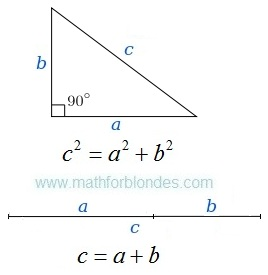Right triangle and two a line segments
How one formula turns into other formula? To see it, we use the law of cosines. We will draw the picture, we will write down conditions, we will execute transformations.Triangle and the law of cosinesRight triangle and the Pythagorean theorem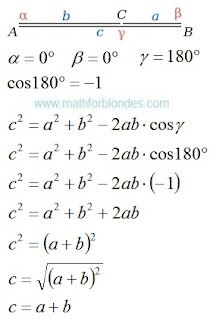The law of cosines and sum of two a line segments
We use the law of cosines and turned the Pythagorean theorem into the sum of two a line segments. Further we will consider an inverse transformation.

If you liked the publication and you want to know more, help me with working on by other publications.

## 2.25.2017

### Law of sines

The law of sines (sine law, sine formula, or sine rule) is an equation relating the lengths of the sides of a triangle (any shape) to the sines of its angles.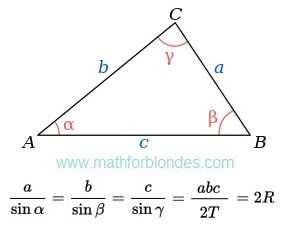The law of sines
Where:
a, b, c - are the lengths of the sides of a triangle;
α, β, γ - are the opposite angles;
T - are the area of triangle;
R - are the radius of the triangle's circumcircle.

How to use this monster? Use dress-making courses. Cut the law of sines on a part.Cut the law of sines on a part
Make a necessary formula of two parts. Use properties of proportions.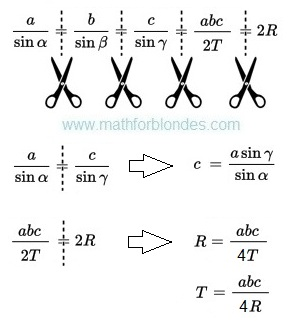Example of use of the law of sine

## 2.13.2017

### Transformations of trigonometric functions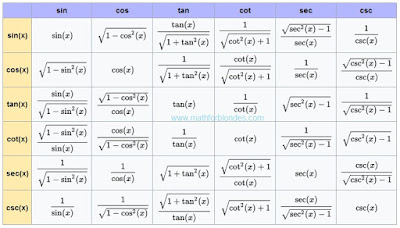Transformations of trigonometric functions
This table shows how one trigonometric functions can be transformed to other trigonometric functions. Sin, cos, tan, cot, sec, csc - all these functions can be transformed.

## 2.10.2017

### Number to the power minus one

What to do if number to the power minus one? Write down this number in a fraction denominator. Here several examples with numbers to the power minus one.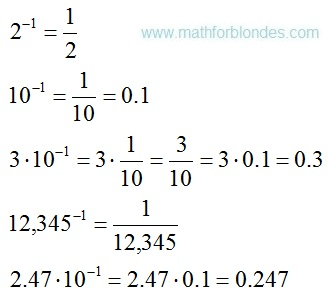Number to the power minus one
Negative exponents of numbers mean that these numbers are in a fraction denominator.Negative exponents

### Trigonometrical circle of a tangent

Last time I drew for you a unit circle of cotangent. Now you can look at a trigonometrical circle of tangent.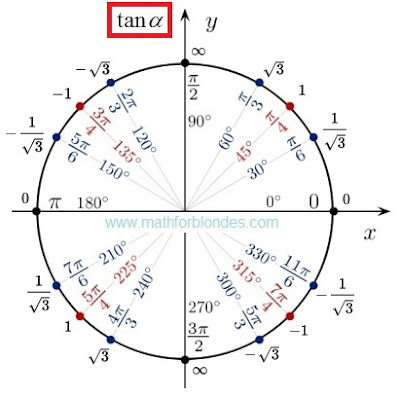Trigonometrical circle of a tangent
There is no sense here. There are angles and values of tangents. If you want to understand sense of a tangent and cotangent, look here.